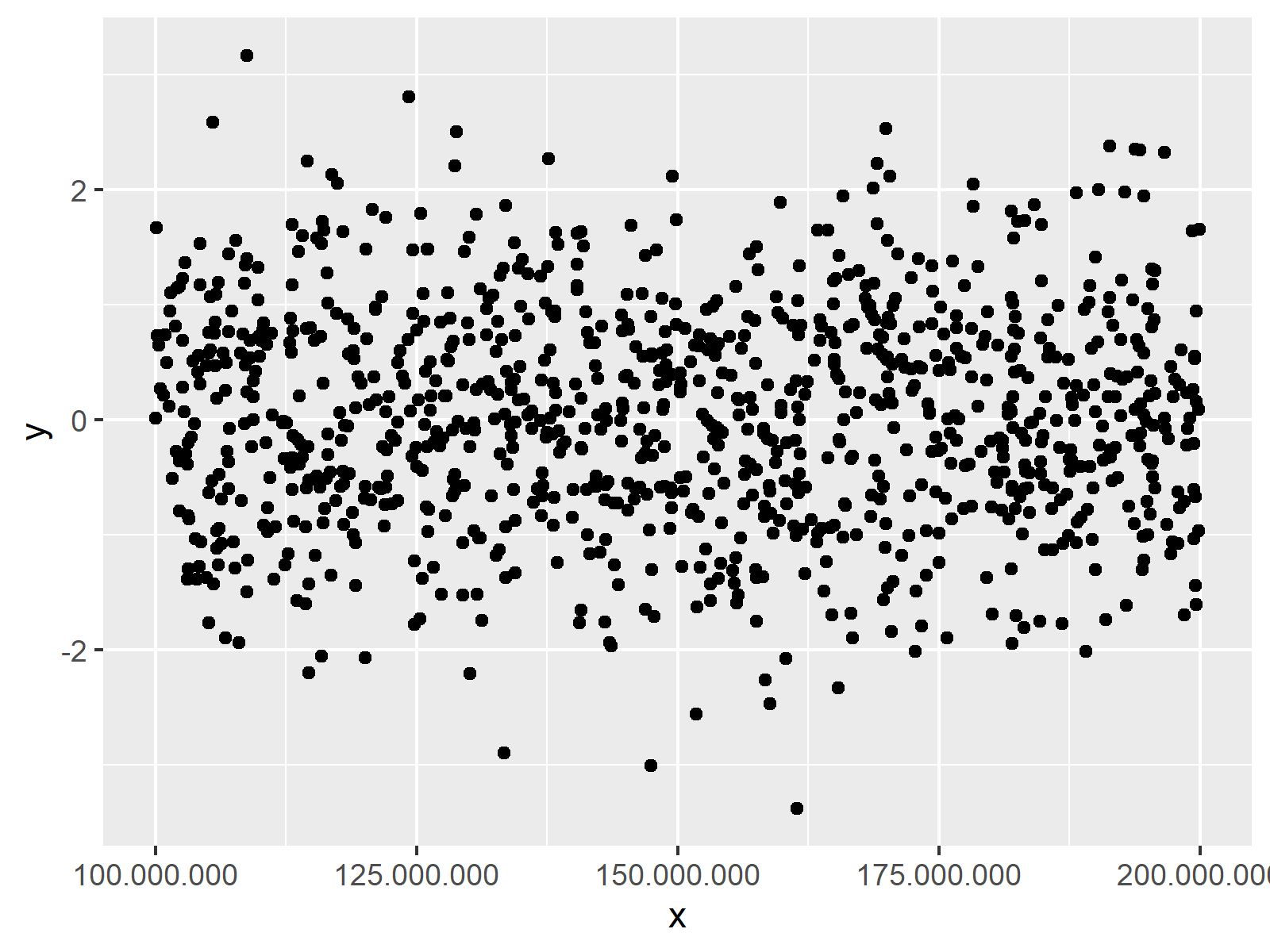# Change Formatting of Numbers of ggplot2 Plot Axis in R (Example)

In this tutorial you’ll learn how to modify the formatting of axis values in a ggplot2 graph in R programming.

The tutorial is structured as follows:

Here’s the step-by-step process.

## Creation of Exemplifying Data

In this R tutorial, I’ll show two examples for the formatting of axis numbers in a ggplot2 plot. Both of these examples will be based on the following example data:

```set.seed(1489)                             # Set seed
data <- data.frame(x = runif(1000,         # Create example data
100000000,
200000000),
y = rnorm(1000))```

Our example data is a data.frame consisting of 1000 rows and two columns x and y. Both variables contain random numeric values.

For this tutorial, we’ll also have to install and load the ggplot2 and scales packages. The ggplot2 package is needed in order to plot our data and the scales package is needed to change the numbers of our plot axes.

```install.packages("ggplot2")                # Install and load packages
library("ggplot2")

install.packages("scales")
library("scales")```

Now, we can draw a scatterplot with the ggplot2 package as follows:

```ggp <- ggplot(data, aes(x, y)) +           # Create basic ggplot2 plot
geom_point()
ggp```Figure 1: Default ggplot2 Scatterplot.

Figure 1 shows the output of the previous R code: A graphic with x-axis values formatted in scientific notation. In the following, you’ll learn how to modify these axis numbers…

## Example 1: Disable Scientific Notation of ggplot2 Axis

Example 1 shows how to disable scientific notation in a ggplot2 plot in R. Have a look at the following R syntax and the resulting graph:

```ggp +                                      # Modify formatting of axis
scale_x_continuous(labels = comma)```Figure 2: Disable Scientific Notation of ggplot2 Plot Axis.

As you can see in Figure 2, we removed the scientific notation of the x-axis. The numbers are fully shown and after every third digit you can see a comma. We did that by applying the scale_x_continuous function in combination with the comma function of the scales package.

## Example 2: Exchange Decimal Point / Comma of ggplot2 Axis

Let’s assume that we want to change the decimal rule from point to comma (this is typically done in Europe). Then we can use the comma_format function of the scales package as shown below:

```ggp +                                      # Change decimal comma / point
scale_x_continuous(labels = comma_format(big.mark = ".",
decimal.mark = ","))```Figure 3: Replace Comma with Point in ggplot2 Plot Axis.

Figure 3 shows our updated graph with commas instead of points.

## Video & Further Resources

Do you want to know more about plotting data in R? Then you might want to have a look at the following video of my YouTube channel. In the video, I illustrate the examples of this tutorial in RStudio.

Please accept YouTube cookies to play this video. By accepting you will be accessing content from YouTube, a service provided by an external third party.If you accept this notice, your choice will be saved and the page will refresh.

In addition, you may have a look at the other tutorials on https://www.statisticsglobe.com/. Some tutorials about graphics in R can be found here.

You learned in this article how to customize axis tick marks in a ggplot2 image in R. If you have additional questions, let me know in the comments.

Subscribe to the Statistics Globe Newsletter